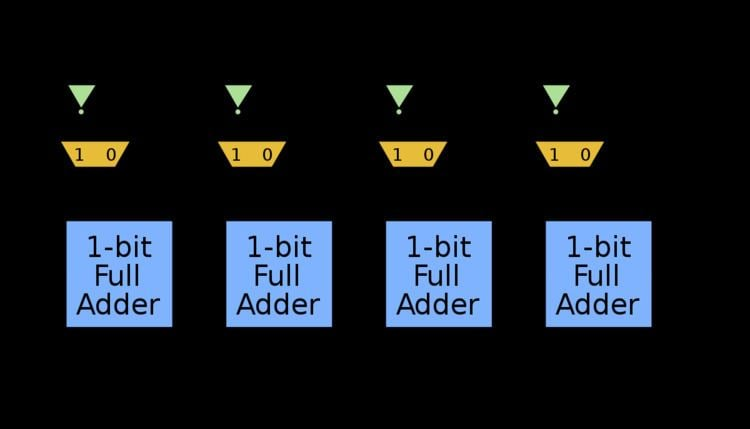Updated on
Covid-19In digital circuits, an adder–subtractor is a circuit that is capable of adding or subtracting numbers (in particular, binary). Below is a circuit that does adding or subtracting depending on a control signal. It is also possible to construct a circuit that performs both addition and subtraction at the same time.

## Construction

Having an n-bit adder for A and B , then S = A + B . Then, assume the numbers are in two's complement. Then to perform B A , two's complement theory says to invert each bit with a NOT gate then add one. This yields S = B + A ¯ + 1 , which is easy to do with a slightly modified adder.

By preceding each A input bit on the adder with a 2-to-1 multiplexer where:

• Input 0 ( I 0 ) is A
• Input 1 ( I 1 ) is A ¯
• that has control input D that is also connected to the initial carry, then the modified adder performs

• addition when D = 0 , or
• subtraction when D = 1 .
• This works because when D = 1 the A input to the adder is really A ¯ and the carry in is 1 . Adding B to A ¯ and 1 yields the desired subtraction of B A .

A way you can mark number A as positive or negative without using a multiplexer on each bit is to use a XOR (Exclusive OR) gate to precede each bit instead.

• First input to the XOR gate is the actual input bit
• Second input to the XOR gate for each is the control input D
• This produces the same truth table for the bit arriving at the adder as the multiplexer solution does since the XOR gate output will be what the input bit is when D = 0 and the inverted input bit when D = 1 .

## Role in the arithmetic logic unit

Adders are a part of the core of an arithmetic logic unit (ALU). The control unit decides which operations an ALU should perform (based on the op code being executed) and sets the ALU operation. The D input to the adder–subtractor above would be one such control line from the control unit.

The adder–subtractor above could easily be extended to include more functions. For example, a 2-to-1 multiplexer could be introduced on each B i that would switch between zero and B i ; this could be used (in conjunction with D = 1 ) to yield the two's complement of A since A = A ¯ + 1 .

A further step would be to change the 2-to-1 mux on A to a 4-to-1 with the third input being zero, then replicating this on B i thus yielding the following output functions:

• 0 (with the both A i and B i input set to zero and D = 0 )
• 1 (with the both A i and B i input set to zero and D = 1 )
• A (with the B i input set to zero)
• B (with the A i input set to zero)
• A + 1 (with the B i input set to zero and D = 1 )
• B + 1 (with the A i input set to zero and D = 1 )
• A + B
• A B
• B A
• A ¯ (with A i set to invert; B i set to zero; and D = 0 )
• A (with A i set to invert; B i set to zero; and D = 1 )
• B ¯ (with B i set to invert; A i set to zero; and D = 0 )
• B (with B i set to invert; A i set to zero; and D = 1 )
• By adding more logic in front of the adder, a single adder can be converted into much more than just an adder—an ALU.

Topics